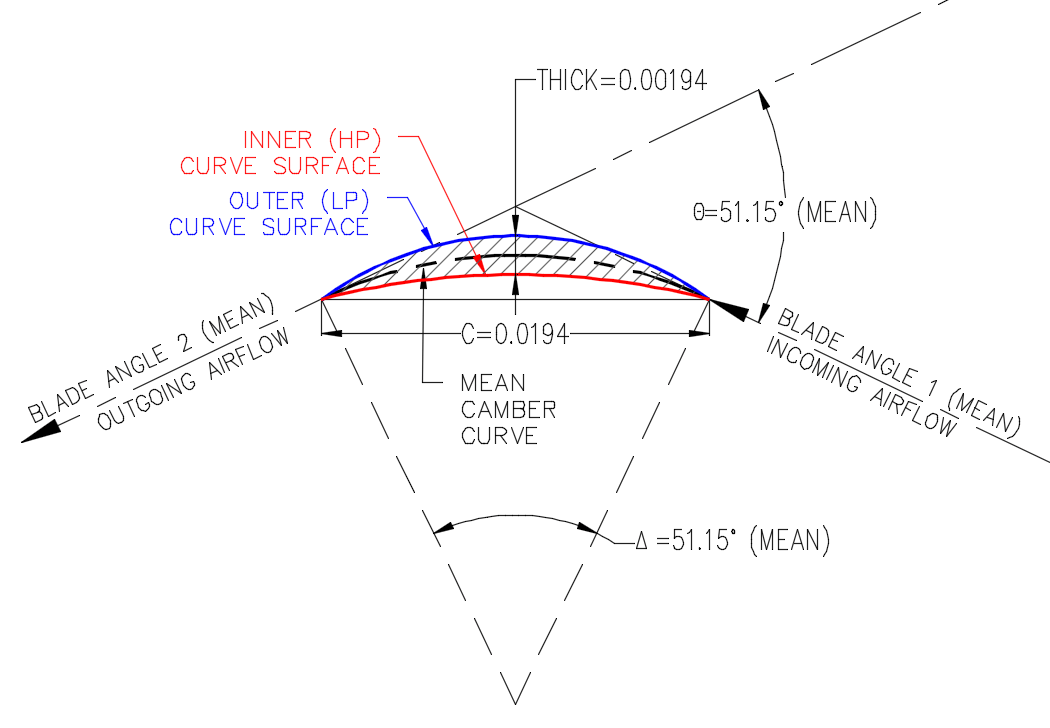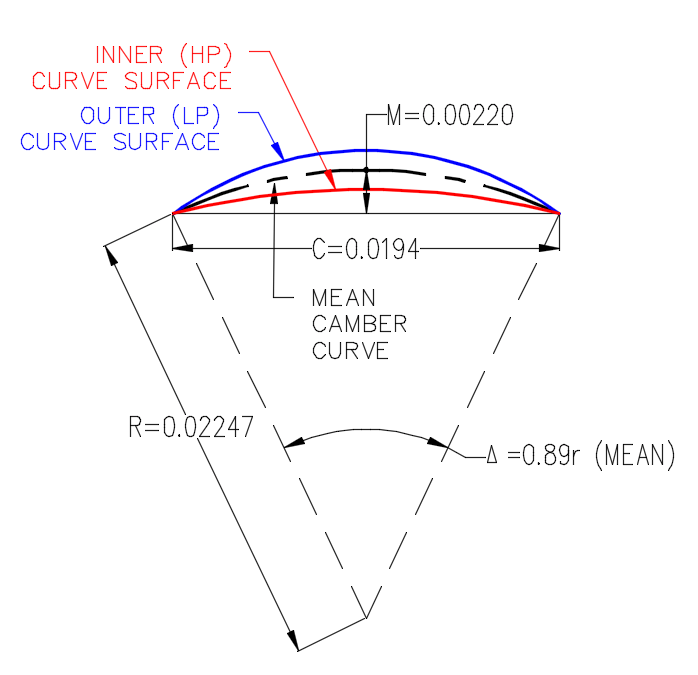In order to determine how fast the High-Pressure Compressor can safely spin, we need to determine how much stress the blades and hubs are experiencing. If I throw something together and build the CFD (computational fluid dynamics) and FEA (finite entity analysis) models, I would end up with a complete overhaul to the design, and have to repeat the build processes. By employing some basic 2D calculations of selected stress concentrations which the HP compressor will experience can save considerable revision time.

Stress is defined as Force/Area. One such stress that needs to be determined is how much stress is acting on the root of the compressor blade. I tried to approximate the area with a few coefficients, but unfortunately, as the blades grow in size and camber, the approximations lose considerable accuracy.

What we can do is to approximate the blade inner and outer curve parameters, calculate the areas under each, and then subtract these to get the net result thereof. If you are using CAD, such as AutoCAD, you can query radii, areas, and centroid parameters graphically. However if you are generating the shapes using CAD parameters, or linked Excel tables, then you are going to have to do some math.

## What we know

From our basic blade flow calculations, we know the centerline (mean camber line) chord length and camber angle of each blade, from root to tip. We also know that the blade thickness factor is 0.1, which indicates that the maximum blade thickness is 10% of the chord length.

Note: To avoid confusion of mean camber line with other references to the mean line design of the flow cross section, I’ll refer to the mean camber line as the centerline of blade (c/l).## What we need to determine

The equations we will use are basic trigonometry relationships in a circular arc. We would be wise to use some calculus to determine these, but trig will be easier in a spreadsheet or CAD parameter field.

Mid Ordinate: M = R(1-Cos(Θ/2))

Camber or Delta angle: Θ = 4*ATan(2 * M/C)

Area under the curve: Area = R^2/2 * (Θ – Sin(Θ))

Note: Area is the area between the curve and the chord.

### symbols will include:

M / Mo / Mi / Mcl = Mid-Ordinate and subscripts for outer, inner, and centerline curves

Θ / Θo / Θi = Delta or Camber angle, in radians, with subscripts for outer and inner curves

AREA = Net area between curves

AREAo / AREAi = Area under respective curves, with subscripts for outer and inner curves

## C/l Curve Calculations

Our first step is to determine the radius and mid-ordinate of the c/l curve.

Why the mid-ordinate you may ask. Because, it is easiest to jump to the inner and outer curves as we already know the offset from the centerline at the thickest point. That would approximately be half the thickness of the blade.R =  C/(2*Sin(Θ/2))

• Rcl = 0.0194 / (2*Sin(0.89274/2)) = 0.02247m

M = R(1-Cos(Θ/2))

• Mcl = 0.02247*(1–Cos(0.89274/2)) = 0.00220m

## Outer and Inner Curve

As mentioned easrlier, if the blade centerline lies in the middle of the inner and outer curves, then the offset between these is 1/2 the thickness.The following calculations are for the outer curve, with subscript _o.

Mid Ordinate:

Mo = Mcl + 0.5*thickness

• Mo = 0.00220 + 0.5*0.00194 = 0.00317m

Using the Chord length and Mid Ordinate, we can determine the remaining values.

Camber Angle:

Θ = 4*ATan(2 * M/C)

• Θo = 4 * ATan(2 * 0.00317/0.0194) = 1.26345 rad

R =  C/(2*Sin(Θ/2))

• Ro = 0.0194/(2 * Sin(1.26345/2)) = 0.01643m

For the inner curve, with subscript _i, we’d subtract the half thickness instead of adding, then repeat the remaining calculations.

Mi = 0.00220 – 0.5*0.00194 = 0.00123m

• Θi = 4 * ATan(2 * 0.00123/0.0194) = 0.50452r
• Ri = 0.0194/(2 * Sin(0.50452/2)) = 0.03886m

Now that we have Radius and Camber, we can determine the area under the curve.Area = R^2/2 * (Θ – Sin(Θ))

• AREAo = 0.01643^2 /2 * (1.26345 – Sin(1.26345) = 0.000042 m^2

Now, we can repeat the entire process for the inner curve and get it’s area:

• AREAi = 0.03886^2 /2 * (0.50452 – Sin(0.50452) = 0.000016m^2
• Area of blade cross section = AREAo – AREAi
• Area = 0.000042 – 0.000016 = 0.000026m^2

## Conclusion

That seems like a lot of work that some calculus could simplify; very true. However if you are working in Excel or CAD parameters, you need something that’s algebraic (plus I’m not the best at Calculus).

Our old coefficient estimation of this curve was 0.000031m^2, which is about 20% off. That difference applied into three factors of the principle stress calculations should be enough to cause considerable uncertainty. With a safety factor of 3+, 20% starts eating up our usable design room quickly. If each stress estimation is out by 20%, the design stability is overestimated tremendously.

I’ll bring other ways of determining some of this information, as well as centroid calculation, second moment of area, bending moment, stress calculations and more. Keep checking back at Engineering Notes.

### Disclaimer

While the standard arc trigonometry equations are real, the application for applying these for compressor blade root area cross sections is approximate. If you simply need the trig information for circular arcs, then you are set. If you are applying these factors to DCA airfoil calculations, which can vary shape somewhat, be aware that these are only close estimations intended to get you running quickly.

## The entire trigonometry equation for Excel

If you want to simplify things a bit, the following is the whole enchilada, in one equation. You can paste that into Excel if you like, and it only needs you to supply the Chord, and the inner and outer Mid Ordinates.

Area = (((C/(2*SIN(ATAN(2*M)/C)))^2)/2)*((4*ATAN((2*M)/C))-SIN(4*ATAN((2*M)/C)))